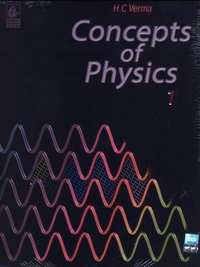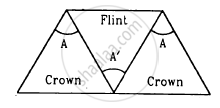Share

# HC Verma solutions for Class 12 Concepts of Physics 1 chapter 20 - Dispersion and Spectra [Latest edition]

Textbook page

#### Chapters## Chapter 20: Dispersion and Spectra

### HC Verma solutions for Class 12 Concepts of Physics 1 Chapter 20 Dispersion and Spectra Short Answers [Page 441]

Short Answers | Q 1 | Page 441

The equation $\omega = \frac{\mu_u - \mu_r}{\mu - 1}$ was derived for a prism having small refracting angle. Is it also valid for a prism of large refracting angle? Is it also valid for a glass slab or a glass sphere?

Short Answers | Q 2 | Page 441

Can the dispersive power $\omega = \frac{\mu_u - \mu_r}{\mu - 1}$ be negative? What is the sign of ω if a hollow prism is immersed into water?

Short Answers | Q 3 | Page 441

If three identical prisms are combined, is it possible to pass a beam that emerges undeviated? Undispersed?

Short Answers | Q 4 | Page 441

"Monochromatic light should be used to produce pure spectrum". Comment on this statement.

Short Answers | Q 5 | Page 441

Does focal length of a lens depend on the colour of the light used? Does focal length of a mirror depend on the colour?

Short Answers | Q 6 | Page 441

Suggest a method to produce a rainbow in your house.

### HC Verma solutions for Class 12 Concepts of Physics 1 Chapter 20 Dispersion and Spectra MCQ [Pages 441 - 442]

MCQ | Q 1 | Page 441

The angular dispersion produced by a prism ___________ .

• increases if the average refractive index increases

• increases if the average refractive index decreases

• remains constant whether the average refractive index increases or decreases

• has no relation with average refractive index

MCQ | Q 2 | Page 442

If a glass prism is dipped in water, its dispersive power ___________ .

• increases

• decreases

• does not change

• may increase or decrease depending on whether the angle of the prism is less than or greater than 60°

MCQ | Q 3 | Page 442

A prism can produce a minimum deviation δ in a light beam. If three such prisms are combined, the minimum deviation that can be produced in this beam is _______________.

• 0

• δ

MCQ | Q 4 | Page 442

Consider the following two statements :-

(A) Line spectra contain information about atoms.

(B) Band spectra contain information about molecules.

• Both A and B are wrong

• A is correct but B is wrong

• B is correct but A is wrong

• Both A and B are correct

MCQ | Q 5 | Page 442

The focal length of a converging lens are fv and fr for violet and red light respectively.

• fv > fr

• fv = fr

• fv < fr

• Any of the three is possible depending on the value of the average refractive index μ.

### HC Verma solutions for Class 12 Concepts of Physics 1 Chapter 20 Dispersion and Spectra MCQ [Page 442]

MCQ | Q 1 | Page 442

A narrow beam of white light goes through a slab having parallel faces.

(a) The light never splits in different colours

(b) The emergent beam is white

(c) The light inside the slab is split into different colours

(d) The light inside the slab is white

MCQ | Q 2 | Page 442

By properly combining two prisms made of different materials, it is possible to

(a) have dispersion without average deviation

(b) have deviation without dispersion

(c) have both dispersion and average deviation

(d) have neither dispersion nor average deviation

MCQ | Q 3 | Page 442

In producing a pure spectrum, the incident light is passed through a narrow slit placed in the focal plane of an achromatic lens because a narrow slit ___________ .

• produces less diffraction

• increases intensity

• allows only one colour at a time

• allows a more parallel beam when it passes through the lens

MCQ | Q 4 | Page 442

Which of the following quantities related to a lens depend on the wavelength or wavelengths of the incident light?

(a) Power

(b) Focal length

(c) Chromatic aberration

MCQ | Q 5 | Page 442

Which of the following quantities increase when wavelength is increased? Consider only the magnitudes.

(a) The power of a converging lens

(b) The focal length of a converging lens

(c) The power of a diverging lens

(d) The focal length of a diverging lens

### HC Verma solutions for Class 12 Concepts of Physics 1 Chapter 20 Dispersion and Spectra [Pages 442 - 443]

Q 1 | Page 442

A flint glass prism and a crown glass prism are to be combined in such a way that the deviation of the mean ray is zero. The refractive index of flint and crown glasses for the mean ray are 1.620 and 1.518 respectively. If the refracting angle of the flint prism is 6.0°, what would be the refracting angle of the crown prism?

Q 2 | Page 442

A certain material has refractive indices 1.56, 1.60 and 1.68  rfor red, yellow and violet lightespectively. (a) Calculate the dispersive power. (b) Find the angular dispersion produced by a thin prism of angle 6° made of this material.

Q 3 | Page 442

The focal lengths of a convex lens for red, yellow and violet rays are 100 cm, 98 cm and 96 cm respectively. Find the dispersive power of the material of the lens.

Q 4 | Page 442

The refractive index of a material changes by 0.014 as the colour of the light changes from red to violet. A rectangular slab of height 2.00 cm made of this material is placed on a newspaper. When viewed normally in yellow light, the letters appear 1.32 cm below the top surface of the slab. Calculate the dispersive power of the material.

Q 5 | Page 442

A thin prism is made of a material having refractive indices 1.61 and 1.65 for red and violet light. The dispersive power of the material is 0.07. It is found that a beam of yellow light passing through the prism suffers a minimum deviation of 4.0° in favourable conditions. Calculate the angle of the prism.

Q 6 | Page 442

The minimum deviations suffered by, yellow and violet beams passing through an equilateral transparent prism are 38.4°, 38.7° and 39.2° respectively. Calculate the dispersive power of the medium.

Q 7 | Page 442

Two prisms of identical geometrical shape are combined with their refracting angles oppositely directed. The materials of the prisms have refractive indices 1.52 and 1.62 for violet light. A violet ray is deviated by 1.0° when passes symmetrically through this combination. What is the angle of the prisms?

Q 8 | Page 442

Three thin prisms are combined as shown in figure. The refractive indices of the crown glass for red, yellow and violet rays are μr, μy and μv respectively and those for the flint glass are μ'r, μ'y and μ'v respectively. Find the ratio A'/A for which (a) there is no net angular dispersion, and (b) there is no net deviation in the yellow ray.Q 9 | Page 443

A thin prism of crown glass (μr = 1.515, μv = 1.525) and a thin prism of flint glass (μr = 1.612, μv = 1.632) are placed in contact with each other. Their refracting angles are 5.0° each and are similarly directed. Calculate the angular dispersion produced by the combination.

Q 10 | Page 443

A thin prism of angle 6.0°, ω = 0.07 and μy = 1.50 is combined with another thin prism having ω = 0.08 and μy = 1.60. The combination produces no deviation in the mean ray. (a) Find the angle of the second prism. (b) Find the net angular dispersion produced by the combination when a beam of white light passes through it. (c) If the prisms are similarly directed, what will be the deviation in the mean ray? (d) Find the angular dispersion in the situation described in (c).

Q 11 | Page 443

The refractive index of a material M1 changes by 0.014 and that of another material M2 changes by 0.024 as the colour of the light is changed from red to violet. Two thin prisms, one made of M1(A = 5.3°) and the other made of M2(A = 3.7°) are combined with their refracting angles oppositely directed. (a) Find the angular dispersion produced by the combination. (b) The prisms are now combined with their refracting angles similarly directed. Find the angular dispersion produced by the combination.

## Chapter 20: Dispersion and Spectra## HC Verma solutions for Class 12 Concepts of Physics 1 chapter 20 - Dispersion and Spectra

HC Verma solutions for Class 12 Concepts of Physics 1 chapter 20 (Dispersion and Spectra) include all questions with solution and detail explanation. This will clear students doubts about any question and improve application skills while preparing for board exams. The detailed, step-by-step solutions will help you understand the concepts better and clear your confusions, if any. Shaalaa.com has the CBSE Class 12 Concepts of Physics 1 solutions in a manner that help students grasp basic concepts better and faster.

Further, we at Shaalaa.com provide such solutions so that students can prepare for written exams. HC Verma textbook solutions can be a core help for self-study and acts as a perfect self-help guidance for students.

Concepts covered in Class 12 Concepts of Physics 1 chapter 20 Dispersion and Spectra are Light Process and Photometry, Total Internal Reflection, Refraction, Ray Optics - Mirror Formula, Reflection of Light by Spherical Mirrors, Refraction Through a Prism, Refraction by a Lens, Snell’s Law, Concave Mirror, Rarer and Denser Medium, Lensmaker's Formula, Thin Lens Formula, Lenses, The Microscope, Some Natural Phenomena Due to Sunlight, Dispersion by a Prism, Combination of Thin Lenses in Contact, Telescope, The Eye, Power of a Lens, Magnification, Refraction at Spherical Surfaces, Principle of Superposition of Waves, Corpuscular Theory, Speed of Light, Coherent and Incoherent Sources and Sustained Interference of Light, Interference of Light Waves and Young’S Experiment, Reflection and Refraction of Plane Wave at a Plane Surface Using Wave Fronts, Huygens Principle, Introduction of Wave Optics, The Validity of Ray Optics, Seeing the Single Slit Diffraction Pattern, The Single Slit, The Doppler Effect, Reflection of a Plane Wave by a Plane Surface, Refraction at a Rarer Medium, Refraction of a Plane Wave, Refraction of Monochromatic Light, Law of Malus, Width of Central Maximum, Polarisation, Resolving Power of Microscope and Astronomical Telescope, Interference, Proof of Laws of Reflection and Refraction Using Huygen'S Principle, Brewster's Law, Plane Polarised Light, Fraunhofer Diffraction Due to a Single Slit, Coherent and Incoherent Addition of Waves.

Using HC Verma Class 12 solutions Dispersion and Spectra exercise by students are an easy way to prepare for the exams, as they involve solutions arranged chapter-wise also page wise. The questions involved in HC Verma Solutions are important questions that can be asked in the final exam. Maximum students of CBSE Class 12 prefer HC Verma Textbook Solutions to score more in exam.

Get the free view of chapter 20 Dispersion and Spectra Class 12 extra questions for Class 12 Concepts of Physics 1 and can use Shaalaa.com to keep it handy for your exam preparation